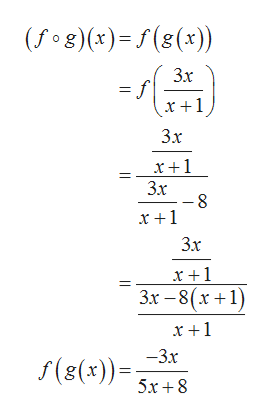# given f(x)= (x)/(x-8) and g(x)= (3x)/(x+1)find (f o g)(x) and state its domain

Question
4 views

given f(x)= (x)/(x-8) and g(x)= (3x)/(x+1)
find (f o g)(x) and state its domain

check_circle

Step 1

From the given information it is required to calculate composition of given two functions.

Step 2

Now, calculate the composition of two functions:help_outlineImage Transcriptionclose(Гоg)(+)-/(«(*)) Зх х+1 Зх х+1 Зx х +1 Зх х+1 Зх — 8(х +1) х+1 —3х S(8(x))= 5х+8 || || fullscreen
Step 3

Domain is the set of possible values for which function is defined.

The composition f...

### Want to see the full answer?

See Solution

#### Want to see this answer and more?

Solutions are written by subject experts who are available 24/7. Questions are typically answered within 1 hour.*

See Solution
*Response times may vary by subject and question.
Tagged in

### Algebra